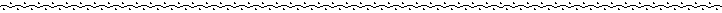Sample Exams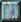Exam I - Fall 1994Exam II - Fall 1994Exam III - Fall 1994Exam I - Spring 1995Exam II - Spring 1995Exam III - Spring 1995Exam I - Spring 1996Exam II - Spring 1996Exam III - Spring 1996Math 121
Fall 1994
Exam 1

Please write in the blue books provided. When calculations are asked for, show the details of your work. When interpretations or explanations are called for, be clear and concise. You may use a calculator but may not use Minitab on any part of the exam.

1. The following stemplot represents the yearly percentage increases in Dickinson's comprehensive fees over the past 22 years. (3 | 8 means that one year had a percentage increase of 3.8%.)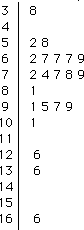1. In what proportion of these years was the percentage increase greater than 10%?
2. The median percentage increase is 7.75%. Without calculating the mean, do you expect it to be greater than the median or less than the median?
3. Is this distribution skewed to the left, skewed to the right, or roughly symmetric?
4. What is the mode of the percentage increases as represented here?

2. Consider the question of whether women tend to pay more for a haircut than do men. Students were asked to report the total cost of their most recent haircut. A total of 17 men and 15 women responded. Their results follow with the amounts recorded in dollars; notice that these values have been ordered.

 men: women: 0.00 4 4.25 6 7 7 8 8 8 10 10 10 10 12 15 15.00 17.00 11.00 12 14 15 15 15 15 15 18 18 20 20 25 25 50

1. Calculate the median haircut price for the men who responded and the median haircut price for the women who responded.
2. The lower and upper quartiles for the men's haircut prices are \$6.50 and \$11.00; the lower and upper quartiles for the women's haircut prices are \$15.00 and \$20.00. Use this information to construct (on the same scale) modified boxplots of the haircut prices for both sexes.
3. Write a few sentences comparing and contrasting the distributions of haircut prices between men and women. Indicate whether the data support the proposition than women tend to pay more for haircuts than do men. Also comment on whether every woman pays more for a haircut than does every man.

3. Suppose that a company employs five men and five women. Construct a hypothetical example which demonstrates that even though the mean salary for men is much higher than the mean salary for women, it is possible for most of the women in the company to earn more than most of the men. List five hypothetical men's salaries and five hypothetical women's salaries for which the following conditions hold:
• the mean salary for men is higher than the mean salary for women
• four of the five lowest salaries in the company belong to men
• four of the five highest salaries in the company belong to women

4. Describe a situation (not taken from your Activity Guide ) in which you would expect a fairly strong association between two variables that do not have a cause-and-effect relationship.

5. In a study of whether a relationship exists between a child's aptitude and the age at which he/she first speaks, researchers recorded the age (in months) of a child's first speech and the child's score on an aptitude test. These data for these 21 children follow:

 child age score child age score 1 2 3 4 5 6 7 8 9 10 11 15 2 10 9 15 20 18 11 8 20 7 95 71 83 91 102 87 93 100 104 94 113 12 13 14 15 16 17 18 19 20 21 9 10 11 11 10 12 42 17 11 10 96 83 84 102 100 105 57 121 86 100

The least squares line for predicting aptitude score from age at first speech turns out to be score = 110 - 1.13 * age; the value of the correlation coefficient is -0.640. The following scatterplot displays this relationship.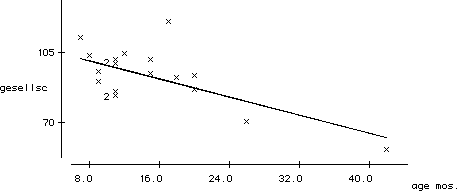1. What proportion of the variability in aptitude scores is explained by the least squares line with age at first speech?
2. What would the least squares line predict for the aptitude score of a child who first spoke at 20 months?
3. Calculate the residual for child 6.
4. Judging from the scatterplot, which child has the largest (in absolute value) residual? What is unusual about this child?
5. Which child has the smallest fitted value?
6. Which child seems to be the most influential observation?

6. Suppose that college students are asked to identify their preferences in political affiliation (Democrat, Republican, or Independent) and in ice cream (chocolate, vanilla, or strawberry). Suppose that their responses are represented in the following two-way table (with some of the totals left for you to calculate):

 chocolate Democrat Republican Independent vanilla strawberry total 26 43 13 82 45 12 8 65 9 13 4 68 25 173

1. What proportion of the respondents prefer chocolate ice cream?
2. What proportion of the respondents are Independents?
3. What proportion of Independents prefer chocolate ice cream?
4. What proportion of those who prefer chocolate ice cream are Independents?
5. Study the following segmented bar graph displaying the conditional distributions of ice cream preference among the three political affiliations. Write a few sentences commenting on whether the (fictitious) data reveal any relationship between political affiliation and ice cream preference.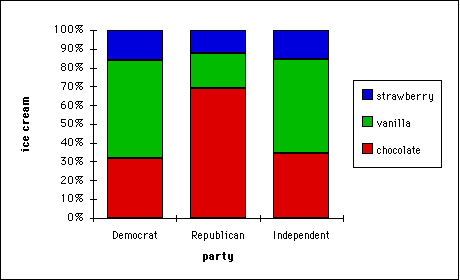Math 121
Fall 1994
Exam 2

Please write in the blue books provided. Show the details of your calculations. You may use a calculator, your Activity Guide (including the table of standard normal probabilities), and your homework solutions on this exam.

1. A 1989 sample of 130 college women who visited a gynecologist at a particular university in the northeastern U.S. indicated that 113 were sexually experienced.
1. Assuming that these women were a simple random sample from the population of all women at that university, calculate a 95% confidence interval for the proportion of the population who are sexually active.
2. Would the interval have been wider, narrower, or the same width if 520 women had been sampled? (You need not perform any calculation.) Explain.
3. Would the interval have been wider, narrower, or the same width if it had turned out that 73 of the 130 women in the sample had been sexually experienced? (You need not perform any calculation.) Explain.
4. Write a sentence interpreting what this interval means (as if this were a random sample).
5. Do you think it is reasonable to assume that these women form a random sample? Explain.

2. Suppose you want to estimate the proportion of American households that own a cat.
1. In order to estimate this proportion to within � .05 with 90% confidence, how many households would you need to sample? (Supply your own guess concerning the proportion in determining the necessary sample size; identify this guess as such.)
2. If you want to estimate this proportion to within � .10 with 90% confidence, would you need to sample more or fewer households than in (a)? (You need not perform the calculation.) Explain.
3. If you want to estimate this proportion to within � .02 with 95% confidence, would you need to sample more or fewer households than in (a)? (You need not do the calculation.) Explain.
4. Explain how your answer to (a) would have differed if you wanted to estimate the proportion of all Pennsylvanian households that own a cat to within � .05 with 90% confidence. (You need not perform any calculation.) Explain.

3. Do not perform any calculations to answer the following. Explain your reasoning in each case.
1. Three researchers Alex, Bob, and Chuck independently select random samples from the same population. The sample sizes are 1000 for Alex, 4000 for Bob, and 250 for Chuck. Each researcher constructs a 95% confidence interval forfrom his data. The half-widths of the three intervals are .015, .031, and .062. Match each half-width with its researcher.
2. Two researchers Donna and Eileen each select random samples of size 1000 from different populations and construct 95% confidence intervals for. The half-width of Donna's interval is .030 and the half-width of Eileen's is .025. Given that the sample proportions were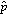=.20 and=.40, match each researcher with her sample proportion.
3. A researcher Fran selects 100 subjects at random from a population, observes 50 successes, and calculates five confidence intervals. The confidence levels are 80%, 90%, 95%, 98%, and 99%, and the five intervals are (.402,.598), (.371,.629), (.418,.582), (.436,.564), and (.384,.616). Match each interval with its confidence level.

4. Suppose that weights of bags of potato chips coming from a factory follow a normal distribution with mean 12.8 ounces and standard deviation .6 ounces.
1. What proportion of bags weigh more than 12 ounces?
2. What proportion of bags weigh between 13 and 14 ounces?
3. Determine the weight such that 12.5% of the bags weigh more than that weight.
4. If the manufacturer wants to keep the mean at 12.8 ounces but adjust the standard deviation so that only 1% of the bags weigh less than 12 ounces, how small does he/she need to make that standard deviation?

5. Suppose that 80% of all Pennsylvania residents eat turkey on Thanksgiving. Suppose further that you plan to select a simple random sample of 300 Pennsylvania residents and to determine the proportion of them who eat turkey on Thanksgiving.
1. Is 80% a parameter or a statistic? What symbol have we used to represent it?
2. According to the Central Limit Theorem, how would the sample proportion who eat turkey on Thanksgiving vary from sample to sample?
3. Determine the probability that less than three-fourths of the sample eat turkey on Thanksgiving.
4. Would the answer to (c) be smaller, larger, or the same if a sample size of 800 was used? (You need not perform the calculation.) Explain.
5. One can show that in this context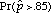� 0.15. Write a sentence or two explaining for a layperson what this statement means.Math 121
Fall 1994
Exam 3

Please write in the blue books provided. When calculations are asked for, show the details of your work. When interpretations or explanations are called for, be clear and concise. You may use a calculator but may not use Minitab on any part of the exam. You may use your Activity Guide and your homework solutions.

1. Suppose that you want to test whether the mean age of a bride in Cumberland County differs from 30 years of age. You gather sample data on 24 marriage licenses and find the following ages for the brides:

 22 32 50 25 33 27 45 47 30 44 23 39 24 22 16 73 27 36 24 60 26 23 28 36

The mean of these ages is 33.83 and the standard deviation of these ages is 13.56.

1. Are the 33.83 and 13.56 parameters or statistics? Indicate the symbols that we have used to represent them.
2. Record the null and alternative hypotheses for the test of significance to address the issue stated above.
3. Calculate the test statistic for this test.
4. Use the appropriate table to calculate the test's p-value as accurately as you can.
5. Write a sentence or two describing and explaining your conclusion about whether the mean age of a bride in Cumberland County differs from 30 years of age.

2. An article appearing in the October 4, 1994 issue of The Harrisburg Evening-News reported that Judge Lance Ito (who is trying the O.J. Simpson murder case) had received 812 letters from around the country on the subject of whether to ban cameras from the courtroom. Of these 812 letters, 800 expressed the opinion that cameras should be banned.
1. Use this sample information to conduct a test of significance of whether more than 95% of all American adults feel that cameras should be banned from the courtroom.
2. Is the test result statistically significant at the .01 level?
3. List the assumptions required for the significance test procedure to be valid in this situation. Comment on whether the assumptions seem to be satisfied.

3. Some researchers wanted to investigate whether the proportion of college students who drink alcohol decreased between 1982 and 1991. They analyzed data from two national studies. In a national study conducted in 1982, 4324 of a sample of 5252 college students said that they drank alcohol. In a similar national study conducted in 1991, 3820 of a sample of 4845 college students said that they drank alcohol.
1. What proportion of the 1982 sample drank alcohol? What proportion of the 1991 sample drank alcohol?
2. State the null and alternative hypotheses for testing the researchers' conjecture in both symbols and words.
3. Calculate the test statistic and the p-value of the test.
4. Is the decrease in sample proportions statistically significant at the .02 level? Explain.

These national studies also asked students more specific questions about their drinking habits. Students were asked whether they have gotten into fights after drinking and whether they have had trouble with the law after drinking. The following table summarizes the sample results and also reports the test statistic of the significance test of whether the population proportions differ between 1982 and 1991.

 1982 sample 1991 test statistic sample 11.6% 17.2% -7.205 4.4% 7.6% -6.115

5. Write a few sentences summarizing the results of these two tests and the test that you performed concerning abstention from drinking alcohol.

4. Suppose that 41 Dickinson students are asked to measure the length of one of their feet. Suppose that the sample mean foot length turns out to be 23.4 centimeters and that the sample standard deviation of the foot lengths turns out to be 5.1 centimeters.
1. Find a 96% confidence interval for µ, the mean foot length among all Dickinson students.
2. Would you expect about 96% of all Dickinson students to have foot lengths within this interval? Explain.
3. If you had found a 90% confidence interval for µ, how would it have differed from the 96% confidence interval?
4. If the sample had included 141 students (and all else had turned out the same), how would the confidence interval have been affected?
5. If the sample standard deviation had turned out to be 3.7 centimeters (and all else had turned out the same), how would the confidence interval have been affected?
6. If the sample mean had turned out to be 25.4 centimeters (and all else had turned out the same), how would the confidence interval have been affected?

5. Use the t-table to find (as accurately as possible):
1. the critical value t * for a 70% confidence interval based on 27 degrees of freedom
2.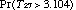
3.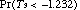4.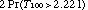6. "The underlying principle of all statistical inference techniques is that one uses sample statistics to learn something (i.e., to infer something) about population parameters ." Convince me that you understand this statement by writing a short paragraph describing a situation in which you might use a sample statistic to infer something about a population parameter. Clearly identify the sample, population, statistic, and parameter in your example. Be as specific as possible. Do not use any example which appears in the Activity Guide.Math 121
Spring 1995
Exam 1

Please write in the blue books provided. When calculations are asked for, show the details of your work. When interpretations or explanations are called for, be clear and concise. You may use a calculator but may not use Minitab on any part of the exam. There are four questions, worth 25 points each, so please budget your time appropriately.

1. The following stemplot represents the yearly percentage increases in Dickinson's comprehensive fees over the past 22 years. (3 | 8 means that one year had a percentage increase of 3.8%.)1. Calculate the median of these percentage increases.
2. The lower quartile is 6.7 and the upper quartile is 9.7. Use this information to test for outliers and then to construct a modified boxplot of the distribution of percentage increases.
3. Write a few sentences commenting on key features of the distribution of percentage increases.

2. Consider the following data dealing with Broadway shows:

show receipts % capacty
Angels in America: Millennium Approaches \$326,121 90.0
Blood Brothers \$154,064 54.5
Cats \$346,723 70.7
Crazy for You \$463,377 85.5
Falsettos \$86,864 39.8
Fool Moon \$163,802 54.2
The Goodbye Girl \$429,158 79.5
Guys and Dolls \$457,087 76.7
Jelly's Last Jam \$253,951 61.6
Kiss of the Spider Woman \$406,498 91.5
Les Miserables \$481,973 91.0
Miss Saigon \$625,804 95.0
Phantom of the Opera \$674,609 101.7
Shakespeare for my Father \$78,898 72.8
The Sisters Rosensweig \$340,862 98.5
Someone Who'll Watch over Me \$73,903 41.7
Tommy \$590,334 86.0
The Will Rogers Follies \$265,561 64.9

The following scatterplot reveals the relationship between receipts and attendance as measured by the percentage of the theater's capacity: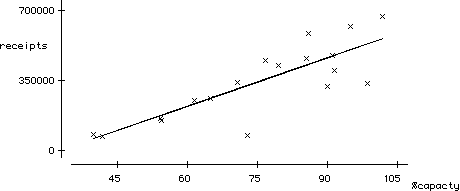 The least squares line turns out to be: receipts = -265,892 + 8118 (% capacity); this line is drawn on the scatterplot.

1. Guess the value of the correlation coefficient between receipts and percentage capacity.
2. What would the regression equation predict for the receipts of a show that filled 80% of its theater's capacity?
3. Calculate the fitted value and residual for Cats .
4. Without doing the calculations, identify which show has the largest (in absolute value) residual.
5. Which show has the smallest fitted value?

3. The following table classifies the living arrangements of American children (under 18 years of age) according to their race and which parent(s) they live with:

 both just mom just dad neither row total 40,842,340 9,017,140 2,121,680 1,060,840 53,042,000 3,833,640 5,750,460 319,470 745,430 10,649,000 4,974,720 2,176,440 310,920 310,920 7,773,000 49,650,700 16,944,040 2,752,070 2,117,190 71,464,000

1. What proportion of the children are black?
2. What proportion of black children live with both parents?
3. What proportion of those who live with both parents are black?

The following segmented bar graph represents the conditional distributions of living arrangements for each race category: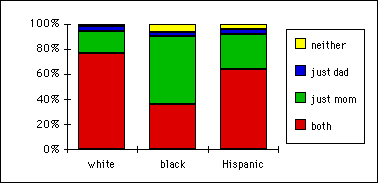4. Comment on any relationship between a child's race and his/her living arrangements as revealed in this graph.

1. Create a set of ten hypothetical exam scores for which the mean is greater than four times the median.
2. The midhinge of a distribution is defined to be the average (mean) of the lower quartile and the upper quartile. The midrange of a distribution is defined to be the average (mean) of the minimum and the maximum. Is the midhinge resistant to outliers? Is the midrange resistant to outliers? Briefly explain.
3. Suppose that 80 of 100 male applicants to a graduate school are accepted, while 60 of 100 female applicants are accepted. Breaking down the applications into the school's two separate programs reveals:
Program A:
72 males accepted out of 80 male applicants

19 females accepted out of 20 female applicants

Program B:

8 males accepted out of 20 male applicants

41 females accepted out of 80 female applicants

Explain why it happens in this example that although each program accepts a higher proportion of females than males, the school as a whole accepts a higher proportion of males than females. (You need not perform the calculations to verify this statement.)
4. Identify a pair of variables (not taken from your Activity Guide) for which you would expect to see a strong correlation but not a cause-and-effect relationship. Suggest an explanation for the association.
5. Suppose that the cases for a study are Dickinson College faculty members. Identify one measurement variable, one non-binary categorical variable, and one binary categorical variable that one could measure on these cases. Identify which type of variable is which.Math 121
Spring 1995
Exam 2

Please write in the blue books provided. Show the details of your calculations. You may use a calculator, your Activity Guide (including the table of standard normal probabilities), and your homework solutions on this exam. Notice that even though every question contains multiple parts, you can answer later parts whether or not you correctly answer earlier parts. Each of the five questions is worth 20 points.

1. In a recent study of Vietnam veterans, researchers found that in a sample of 2101 veterans, 777 had been divorced at least once.
1. Assuming that these women were a simple random sample from the population of all Vietnam veterans, calculate a 90% confidence interval for the proportion of the population who have been divorced at least once.
2. Would the interval have been wider, narrower, or the same width if 1101 veterans had been sampled? (You need not perform any calculation or explain your answer.)
3. Write a sentence interpreting what this interval means (as if this were a random sample).

2. Suppose you want to estimate the proportion of American college students who favor abolishing the penny.
1. In order to estimate this proportion to within � .10 with 92% confidence, how many students would you need to sample? (Supply your own guess concerning the sample proportion in determining the necessary sample size; identify this guess as such.) You need not perform any calculation or explain your answer in (b), (c), and (d) below.
2. If you want to estimate this proportion to within � .05 with 92% confidence, would you need to sample more or fewer students than in (a)?
3. If you want to estimate this proportion to within � .02 with 95% confidence, would you need to sample more or fewer students than in (a)?
4. Explain how your answer to (a) would have differed if you wanted to estimate the proportion of all Pennsylvanian college students who favor abolishing the penny to within � .10 with 92% confidence.

3. Do not perform any calculations to answer the following. Provide a one-sentence explanation of your reasoning in each case.
1. Three researchers Alex, Bob, and Chuck independently select random samples from the same population. The sample sizes are 4000 for Alex, 1000 for Bob, and 250 for Chuck. Each researcher constructs a 95% confidence interval forfrom his data. The half-widths of the three intervals are .015, .031, and .062. Match each half- width with its researcher.
2. Two researchers Donna and Eileen each select random samples of size 1000 from different populations and construct 95% confidence intervals for. The half-width of Donna's interval is .030 and the half-width of Eileen's is .025. Given that the sample proportions were=.20 and=.40, match each researcher with her sample proportion.
3. A researcher Fran selects 100 subjects at random from a population, observes 50 successes, and calculates three confidence intervals. The confidence levels are 90%, 95%, and 99%, and the intervals are (.402,.598), (.371,.629), and (.418,.582). Match each interval with its confidence level.
4. Two researchers George and Henry work together to study a simple random sample of subjects from a population, and they find that the sample proportion is=.60. When they construct a confidence interval based on this sample proportion, George comes up with (.532,.668) while Henry gets (.552,.688). Indicate which interval has to be wrong.

4. Suppose that weights of bags of potato chips coming from a factory follow a normal distribution with mean 12.9 ounces and standard deviation .7 ounces.
1. What proportion of bags weigh more than 12 ounces?
2. Determine the weight such that 12.5% of the bags weigh more than that weight.
3. If the manufacturer wants to keep the mean at 12.9 ounces but adjust the standard deviation so that only 1% of the bags weigh less than 12 ounces, how small does he/she need to make that standard deviation?

5. Consider again the question of whether the home team wins more than half of its games in the National Basketball Association. Suppose that you study a simple random sample of 80 professional basketball games and find that 52 of them are won by the home team.
1. Is 65% (52 / 80) a parameter or a statistic? Explain.
2. Assuming that there is no home court advantage and that the home team therefore wins 50% of its games in the long run, determine the probability that the home team would win 65% or more of its games in a simple random sample of 80 games.
3. Does the sample information (that 52 of a random sample of 80 games are won by the home team) provide strong evidence that the home team wins more than half of its games in the long run? Explain.Math 121
Spring 1995
Exam 3

Please write in the blue books provided. Show the details of your calculations. You may use a calculator, your Activity Guide (including the table of standard normal probabilities), and your homework solutions on this exam. The first question is worth 25 points and the rest are worth 15 points each.

1. A student wanted to assess whether her dog Muffin tends to chase one of her balls more often than the other. She rolled both a blue ball and a red ball at the same time and observed which ball Muffin chose to chase. Repeating this process a total of 96 times, the student found that Muffin chased the blue ball 57 times and the red ball 39 times.
1. In what proportion of the 96 tosses did Muffin chase the blue ball?
2. Is this number from a) a parameter or a statistic? Explain.
3. The student performed a significance test of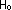:=.5 vs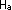:�.5. Write a sentence specifying what the symbol  represents in this context.
4. Calculate the test statistic.
5. Determine the p-value of the test.
6. Write a sentence explaining what the p-value means in this context.
7. Would you reject the null hypothesis at the .10 significance level? At the .05 level? At the .01 level?
8. Indicate the smallest significance levelat which you would reject the null hypothesis.
9. Write a one-sentence conclusion to the student summarizing what the data reveal about whether her dog Muffin tends to chase one of her balls more often than the other.

2. An article appearing in the October 4, 1994 issue of The Harrisburg Evening-News reported that Judge Lance Ito (who is trying the O.J. Simpson murder case) had received 812 letters from around the country on the subject of whether to ban cameras from the courtroom. Of these 812 letters, 800 expressed the opinion that cameras should be banned.
1. Use this sample information to conduct a test of significance of whether more than 95% of all American adults feel that cameras should be banned from the courtroom.
2. Is the test result statistically significant at the .01 level?
3. List the assumptions required for the significance test procedure to be valid in this situation. Comment on whether the assumptions seem to be satisfied.

3. In a study to assess whether aspirin reduces the risk of a pregnant woman developing hypertension, 34 pregnant women were randomly assigned to receive a low dosage of aspirin every day while 31 pregnant women received a placebo every day. Of those in the aspirin group 4 developed hypertension during their pregnancy, compared to 11 of those in the placebo group.
1. Is this study a controlled experiment or an observational study? Explain.
2. Identify the explanatory variable in this study.
3. Identify the response variable in this study.
4. Explain what double-blindness means in the context of this study. Also indicate why it should be used in this study.

4. In a 1984 survey of licensed drivers in Wisconsin, 264 of 1140 women and 214 of 1200 men said that they did not drink alcohol. Conduct the appropriate test of significance to assess whether these sample data provide evidence that Wisconsin women abstain from drinking alcohol at a higher rate than Wisconsin men. Be sure to:
1. specify the null and alternative hypotheses in both symbols and in words,
2. calculate the test statistic,
3. determine the p-value, and
4. write a one-sentence conclusion about the question of interest.

5. A newspaper account of a medical study claimed that the daughters of women who smoked during pregnancy are more likely to smoke themselves. The study surveyed children, asking them if they had smoked in the last year and then asking the mother if she had smoked during pregnancy. Only 4% of the daughters of mothers who did not smoke during pregnancy had smoked in the past year, compared to 26% of girls whose mothers had smoked during pregnancy.
1. Is this study a controlled experiment or an observational study? Explain.
2. What further information do you need to determine if this difference in sample proportions is statistically significant?
3. Describe a scenario in which you suspect that this difference in sample proportions would be statistically significant.
4. Even if the difference in sample proportions is statistically significant, does the study establish that the pregnant mother's smoking caused the daughter's tendency to smoke? Explain.

6. "The underlying principle of all statistical inference techniques is that one uses sample statistics to learn something (i.e., to infer something) about population parameters ." Convince me that you understand this statement by writing a short paragraph describing a situation in which you might use a sample statistic to infer something about a population parameter. Clearly identify the sample, population, statistic, and parameter in your example. Be as specific as possible. Do not use any example which appears in the Activity Guide .Math 121
Exam 1
February 29, 1996

Please write in the blue books provided. When calculations are asked for, show the details of your work. When interpretations or explanations are called for, be clear and concise. You may use a calculator but may not use Minitab on any part of the exam. Please note the point value on each problem and budget your time accordingly.

1. (15 points) The following table lists the running times (in minutes) of the videotape versions of 22 movies directed by Alfred Hitchcock:

film time
The Birds 119
Dial M for Murder 105
Family Plot 120
Foregin Correspondent 120
Frenzy 116
I Confess 108
The Man Who Knew Too Much 120
Marnie 130
North by Northwest 136
Notorious 103
The Paradise Cane 116
Psycho 108
Rear Window 113
Rebecca 132
Rope 81
Shadow of a Doubt 108
Spellbound 111
Strangers on a Train 101
To Catch a Thief 103
Topaz 126
Under Capricorn 117
Vertigo 128

The following stemplot displays the distribution of these running times: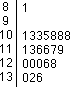1. Calculate the median of these running times.
2. The lower quartile is 108 minutes, and the upper quartile is 120 minutes. Use this information to test for outliers and to construct a modified boxplot of the running times.

2. (20 points) The following table lists the average temperature of a month and the amount of my electricity bill for that month:

 month temp bill month temp bill Apr-91 51 \$41.69 Jun-92 66 \$40.89 May-91 61 \$42.64 Jul-92 72 \$40.89 Jun-91 74 \$36.62 Aug-92 72 \$41.39 Jul-91 77 \$40.70 Sep-92 70 \$38.31 Aug-91 78 \$38.49 Oct-92 * * Sep-91 74 \$37.88 Nov-92 45 \$43.82 Oct-91 59 \$35.94 Dec-92 39 \$44.41 Nov-91 48 \$39.34 Jan-93 35 \$46.24 Dec-91 44 \$49.66 Feb-93 * * Jan-92 34 \$55.49 Mar-93 30 \$50.80 Feb-92 32 \$47.81 Apr-93 49 \$47.64 Mar-92 41 \$44.43 May-93 * * Apr-92 43 \$48.87 Jun-93 68 \$38.70 May-92 57 \$39.48 Jul-93 78 \$47.47

The following scatterplot displys these data. The least squares line is drawn on the scatterplot; the equation of this line is: bill = 55.1 - 0.214 avg temp.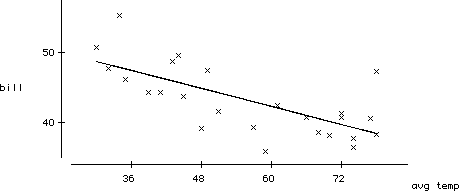1. Estimate the value of the correlation coefficient between electricity bill and average temperature.
2. What would the least squares line predict for the elecricity bill of a month with an average temperature of 60 degrees?
3. Without doing the calculations, identify which month has the largest (in absolute value) residual.
4. Which month has the smallest fitted value?

3. (15 points) The following data address the question of whether percentages of women physicians are changing with time. The table classifies physicians according to their gender and age group.

 under 35 35-44 45-54 55-64 total 93,287 153,921 110,790 80,288 438,286 40,431 44,336 18,026 7,224 110,017 133,718 198,257 128,816 87,512 548,303

1. What proportion of these physicians are women?
2. What proportion of those physicians under age 35 are women?
3. Consider the following segmented bar graph. Comment on whether it reveals any connection between gender and age group. Suggest a plausible explanation for your finding.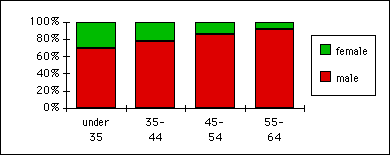4. (10 points) The following table tallies the amounts of 111 withdrawals from an automated teller machine. For example, 17 withdrawals were for the amount of \$20 and 3 were for the amount of \$50; no withdrawals were made of \$40. The total amount withdrawn is \$12,180. Calculate the median and the mode of these withdrawal amounts.

 amount tally \$20 \$50 \$60 \$100 \$120 \$140 \$150 \$160 \$200 \$240 \$250 17 3 7 37 3 8 16 10 8 1 1

5. (20 points) The following boxplots display the distributions of the 1993 governor's salaries according to the state's geographic region of the country. Region 1 is the Northeast, 2 the Midwest, 3 the South, and 4 the West.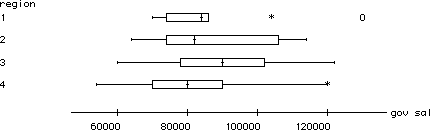

1. Which region has the state with the highest governor's salary?
2. Which region has the state with the highest median governor's salary?
3. Which region has the state with the smallest inter-quartile range of governor's salaries?
4. Estimate the inter-quartile range of the governor's salaries for the Southern states.
5. Estimate the median governor's salary for the Northeatern states.

6. (10 points)
1. Create a set of ten hypothetical exam scores for which the mean is less than 90% of the scores.
2. Explain how it could happen that if one person moves from city A to city B, it is possible for the average (mean) IQ in both cities to increase.

7. (10 points) Suppose that 80 of 100 male applicants to a graduate school are accepted, while 60 of 100 female applicants are accepted. Breaking down the applications into the school's two separate programs reveals:

Program A:
72 males accepted out of 80 male applicants
19 females accepted out of 20 female applicants

Program B:
8 males accepted out of 20 male applicants
41 females accepted out of 80 female applicants

Explain why it happens in this example that although each program accepts a higher proportion of females than males, the school as a whole accepts a higher proportion of males than females. (You need not perform the calculations to verify this statement.)Math 121
Spring 1996
Exam 2

Please write in the blue books provided. Show the details of your calculations. You may use a calculator, your Activity Guide (including the table of standard normal probabilities), and your homework solutions on this exam. Notice that even though many questions contains multiple parts, you can answer later parts whether or not you correctly answer earlier parts. The first two questions are worth 20 points each, and the last four questions are worth 15 points each.

1. In a recent study of a sample of 2101 Vietnam veterans, researchers found that 777 had been divorced at least once.
1. Assuming that these veterans were a simple random sample from the population of all Vietnam veterans, calculate a 90% confidence interval for the proportion of the population who have been divorced at least once.
2. Would the interval have been wider, narrower, or the same width if 4101 veterans had been sampled? Explain your answer without performing the calculation.
3. Can you be sure that your interval contains the sample proportion of Vietnam veterans who had been divorced at least once? Explain briefly.
4. Can you be sure that your interval contains the population proportion of Vietnam veterans who had been divorced at least once? Explain briefly.

2. Suppose that weights of bags of potato chips coming from a factory follow a normal distribution with mean 12.5 ounces and standard deviation .3 ounces.
1. What proportion of bags weigh more than 12 ounces?
2. Determine the weight such that 33% of the bags weigh more than that weight.

3. Do not perform any calculations to answer the following. Provide a one-sentence explanation of your reasoning in each case.
1. Three researchers Alex, Bob, and Chuck independently select random samples from the same population. The sample sizes are 1000 for Alex, 4000 for Bob, and 250 for Chuck. Each researcher constructs a 95% confidence interval forfrom his data. The half-widths of the three intervals are .015, .031, and .062. Match each half-width with its researcher.
2. Two researchers Donna and Eileen work together to study a simple random sample of subjects from a population, and they find that the sample proportion is=.60. When they construct a confidence interval based on this sample proportion, Donna comes up with (.532,.668) while Eileen gets (.552,.688). Indicate which interval has to be wrong.
3. A researcher Fran selects 100 subjects at random from a population, observes 50 successes, and calculates three confidence intervals. The confidence levels are 90%, 95%, and 99%, and the intervals are (.402,.598), (.371,.629), and (.418,.582). Match each interval with its confidence level.

4. Consider a true/false test of 25 questions on which a student guesses randomly at each question. The following histogram presents the results of a simulation of 10,000 such true/false tests. For example, the student got 4 correct on 2 of the 10,000 tests, the student got 5 correct on 15 of the 10,000 tests, the student got 6 correct on 52 of the 10,000 tests, and so on.
1. On what percentage of the 10,000 simulated tests did the student get 7 or fewer correct?
2. On what percentage of the 10,000 simulated tests did the student get between 11 and 14 (including both 11 and 14) of the 25 questions correct?
3. What is the smallest number such that the student got that many or more correct on less than 6% of the 10,000 simulated tests?
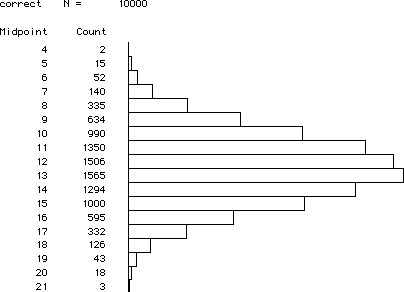5. Suppose you want to estimate the proportion of American college students who favor abolishing the penny.
1. In order to estimate this proportion to within � .05 with 99% confidence, how many students would you need to sample? (Supply your own guess concerning the sample proportion in determining the necessary sample size; identify this guess as such.)
2. If you want to estimate this proportion to within � .08 with 96% confidence, would you need to sample more or fewer students than in (a)? Explain briefly without performing the calculation.

6. Suppose that 80% of all American college students send a card to their mother on Mother's Day. Suppose further that you plan to select a simple random sample of 400 American college students and to determine the proportion of them who send a card to their mother on Mother's Day.
1. Is 80% a parameter or a statistic? Explain briefly.
2. Determine the probability that less than three-fourths of the students sampled send a card to their mother on Mother's Day.Math 121
Spring 1996
Exam 3

Please write in the blue books provided. Show the details of your calculations. You may use a calculator, your book (including the table of standard normal probabilities and the t -table), and your homework solutions on this exam.

1. (25 pts.) A student wanted to assess whether her dog Muffin tends to chase one of her balls more often than the other. She rolled both a blue ball and a red ball at the same time and observed which ball Muffin chose to chase. Repeating this process a total of 96 times, the student found that Muffin chased the blue ball 52 times and the red ball 44 times.
1. In what proportion of the 96 tosses did Muffin chase the blue ball?
2. Is this number from a) a parameter or a statistic? Explain.
3. The student performed a significance test of:=.5 vs:�.5. Write a sentence specifying what the symbolrepresents in this context.
4. Calculate the test statistic.
5. Determine the p-value of the test.
6. Would you reject the null hypothesis at the .10 significance level?
7. Indicate the smallest significance level &181; at which you would reject the null hypothesis.
8. Write a one-sentence conclusion to the student summarizing what the data reveal about whether her dog Muffin tends to chase one of her balls more often than the other.

2. (20 pts.) Many studies have shown that high school students who study a foreign language tend to score higher on the Verbal portion of the Scholastic Aptitude Examination than high school students who do not study a foreign language.
1. Are such studies observational studies or controlled experiments? Explain.
2. Identify the explanatory variable in these studies. Indicate whether it is a categorical or measurement variable. If it is categorical, indicate whether it is also binary.
3. Identify the response variable in these studies. Indicate whether it is a categorical or measurement variable. If it is categorical, indicate whether it is also binary.
4. Can one reasonably conclude from these studies that studying a foreign language causes students to score higher on the Verbal SAT exam? If not, suggest a likely alternative explanation for the finding.

3. (15 pts.) The following lists the word lengths (numbers of letters in the words) for a sample of 26 words from Workshop Statistics :

 10 2 3 7 2 9 4 4 2 1 7 5 2 5 4 5 2 2 9 4 2 5 2 3 4 4

The mean of these word lengths is 4.19, and the standard deviation is 2.45.

1. Form a 90% confidence interval for µ, the mean word length among all words in Workshop Statistics .
2. If the sample size were larger (and the sample mean and standard deviation were the same), how would this confidence interval change?
3. If the sample mean were larger (and the sample size and standard deviation were the same), how would this confidence interval change?
4. A 99% confidence interval for µ turns out to be (2.853, 5.532). What proportion of the 26 words sampled have lengths falling within this interval? Would this answer usually be about 99%? Explain.

4. (15 pts.) Suppose that you want to study the question of college students' having their own credit cards, so you take a random sample of 50,000 college students from around the country. Suppose you find that 24,643 of these students have their own credit card.
1. Does this sample information provide strong evidence that less than half of all American college students have their own credit card? Support your answer with an appropriate test of significance. Provide a one- or two-sentence conclusion.
2. Does this sample information provide evidence that the proportion of all American college students who have their own credit card is very much less than one-half? Support your answer with an appropriate confidence interval. Provide a one- or two-sentence conclusion.

5. (15 pts.) Suppose that 90 of 100 patients who enter hospital A with a particular ailment recover, while 160 of 200 patients who enter hospital B with the same ailment recover. Does this sample information provide strong evidence that hospital A's recovery rate for the disease is significantly higher (at the .05 level) than hospital B's recovery rate? Perform the appropriate test of significance to justify your answer, and provide a one- or two-sentence conclusion.

6. (10 pts.) "The underlying principle of all statistical inference techniques is that one uses sample statistics to learn something (i.e., to infer something) about population parameters ." Convince me that you understand this statement by writing a short paragraph describing a situation in which you might use a sample statistic to infer something about a population parameter. Clearly identify the sample, population, statistic, and parameter in your example. Be as specific as possible, and do not use any example which appears in Workshop Statistics .